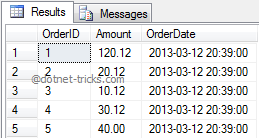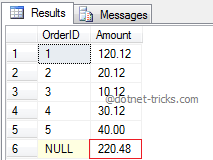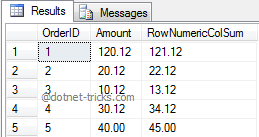# Calculate Running Total, Total of a Column and Row

31 Aug 2022
Intermediate
217K Views

Many times, you required to show information on each transaction and also keep a Running Total and Final Total like GridView in Asp.Net. In this article, I am going to explain, how can you achieve this using SQL Query in a simple and easy way.

Suppose you have the below CustomerOrders table and has the data as shown below:

```CREATE TABLE CustomerOrders
(
OrderID int identity,
Amount Decimal(8,2),
OrderDate SmallDatetime default getdate()
)

Go

INSERT INTO CustomerOrders(Amount) Values(120.12)
INSERT INTO CustomerOrders(Amount) Values(20.12)
INSERT INTO CustomerOrders(Amount) Values(10.12)
INSERT INTO CustomerOrders(Amount) Values(30.12)
INSERT INTO CustomerOrders(Amount) Values(40)

GO

SELECT * FROM CustomerOrders
```## Calculating Running Total

Let's see how to calculate the running total using SQL Query as given below:

``` select OrderID, OrderDate, CO.Amount
,(select sum(Amount) from CustomerOrders
where OrderID <= CO.OrderID)
'Running Total'
from CustomerOrders CO
```## Calculating Final Total

Let's see how to calculate the final total using ROLLUP within SQL Query as given below:

```SELECT OrderID, SUM(Amount) AS Amount
FROM CustomerOrders
GROUP BY OrderID WITH ROLLUP
```## Calculating Total of All Numeric columns in a row

Let's see how to calculate the total of all numeric fields within a row using SQL Query as given below:

```SELECT OrderID, Amount, SUM(OrderID+Amount) AS RowNumericColSum
FROM CustomerOrders
GROUP BY OrderID,Amount
ORDER BY OrderID
```